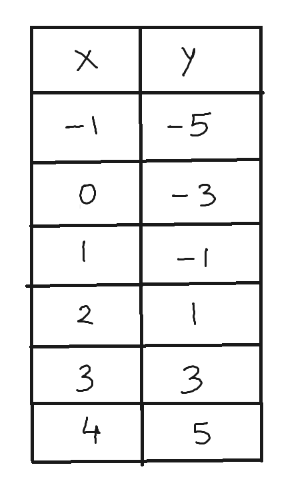# Graph the given equation. First plot the solutions of the equation for the given values of x, and then connect the points with a smooth graph.y = 2x − 3x = −1, 0, 1, 2, 3, 4

Question
24 views

Graph the given equation. First plot the solutions of the equation for the given values of x, and then connect the points with a smooth graph.

 y = 2x − 3 x = −1, 0, 1, 2, 3, 4
check_circle

Step 1

We first make a table for the given values of x.

We p...help_outlineImage Transcriptionclosey -5 - \ - 3 O 2. 3 3 4 5 LO X fullscreen

### Want to see the full answer?

See Solution

#### Want to see this answer and more?

Solutions are written by subject experts who are available 24/7. Questions are typically answered within 1 hour.*

See Solution
*Response times may vary by subject and question.
Tagged in

### Other